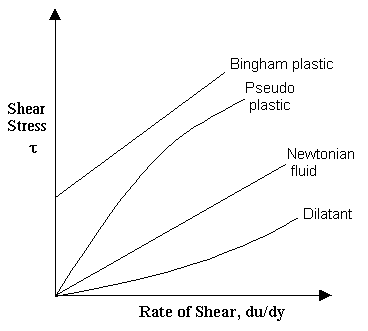### Newtonian and non-Newtonian Fluids

Home -> Lecture Notes -> Fluid Mechanics -> Unit-I

Newtonian fluids:

Fluids which obey the Newton's law of viscosity are called as Newtonian fluids. Newton's law of viscosity is given by

t = mdv/dy

where t = shear stress

m = viscosity of fluid

dv/dy = shear rate, rate of strain or velocity gradient

All gases and most liquids which have simpler molecular formula and low molecular weight such as water, benzene, ethyl alcohol, CCl4, hexane and most solutions of simple molecules are Newtonian fluids.

Non-Newtonian fluids:

Fluids which do not obey the Newton's law of viscosity are called as non-Newtonian fluids.

Generally non-Newtonian fluids are complex mixtures: slurries, pastes, gels, polymer solutions etc.,Various non-Newtonian Behaviors:

Time-Independent behaviors:

Properties are independent of time under shear.

Bingham-plastic: Resist a small shear stress but flow easily under larger shear stresses. e.g. tooth-paste, jellies, and some slurries.

Pseudo-plastic: Most non-Newtonian fluids fall into this group. Viscosity decreases with increasing velocity gradient. e.g. polymer solutions, blood. Pseudoplastic fluids are also called as Shear thinning fluids. At low shear rates(du/dy) the shear thinning fluid is more viscous than the Newtonian fluid, and at high shear rates it is less viscous.

Dilatant fluids: Viscosity increases with increasing velocity gradient. They are uncommon, but suspensions of starch and sand behave in this way. Dilatant fluids are also called as shear thickening fluids.

Time dependent behaviors:Those which are dependent upon duration of shear.

Thixotropic fluids: for which the dynamic viscosity decreases with the time for which shearing forces are applied. e.g. thixotropic jelly paints.

Rheopectic fluids: Dynamic viscosity increases with the time for which shearing forces are applied. e.g. gypsum suspension in water.

Visco-elastic fluids: Some fluids have elastic properties, which allow them to spring back when a shear force is released. e.g. egg white.